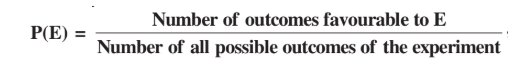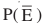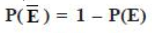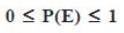Chance and Probability

Chance and Probability - Definition and Example Problems

Chance is the occurrence of events in the absence of any obvious intention or cause. It is, simply, the possibility of something happening. When the chance is defined in mathematics, it is called probability.

Probability is the extent to which an event is likely to occur, measured by the ratio of the favourable cases to the whole number of cases possible.

Mathematically, the probability of an event occurring is equal to the ratio of a number of cases favourable to particular event to the number of all possible cases.

The theoretical probability of an event is denoted as P(E).Assume that we take a coin and toss it, the chances it lands a head is equal to the chances that it lands a tail. Similarly, in any such event, there are equal chances for any of the different cases occurring. For example, in rolling dice, all six numbers are equally likely to be obtained. We also assume that the dice or coin we use is not biased and is fair, i.e. it has not been tampered with an intention to favour a particular outcome.

All the possible outcomes of a random experimentwhen put together is called a sample space.

The each possible results of an event is called an outcome. If an event has only one outcome, then it is called an elementary event

The sum of all the probabilities of eachelementary event is 1. For example, while tossing a coin the two possible outcomes are heads or tails.

P (getting tail) = ½
Now the sum of all possible outcomes will include the probability of heads and probability of tail.
P (tossing a coin) = P (getting head) + P (getting tail)
= ½ + ½
=1
Now, if we must find the probability of an event not occurring. It is denoted with a bar on top.is the probability of event E not occurring and it is called complement of event E. Therefore, we can say that E and are complementary.

P (E) += 1

This also means thatThe probability of an impossible event is 0. This is called an impossible event.
The probability of a sure or certain event is 1. This is called a sure event.
Any probability related to the same event will lie between 0 and 1.example problems
• 1. dice problems

• find the probability of choosing a red ball, blue ball and green ball respectively if a bag contains 5 blue balls, 8 red balls and 10 green balls.

• a. probability of choosing a red ball

• possible outcomes = 23
therefore, the probability of a red ball is 8/23

• b. similarly, the probability of choosing a green ball is 10/23

• c. and the probability of choosing blue ball is 5/23

• 2. pack of card problems

• if one card is drawn from a pack of cards. then what is the probability of a card that will be,

• a. a two

• b. not be a two

• I. there are four 2’s in a pack of cards

• thus, the total number of favourable outcomes = E = 4

total number of possible outcomes =52

therefore, the probability that a 2 will draw from a deck of 52 cards is,

P(E) = 4/52 = 1/13

II. Let T be the event “card drawn is not a 2”

thus, the probability of drawing a card that is not 2 events = T

T = 52 - 4 = 48

therefore,

P(T) = 48/52 = 12/13

an alternate way to find the same solution is,

P(T) = P(E) - 1 = 1 - 1/13 = 12/13

probability is an easy chapter, and thus one must try and get maximum scores. above we have discussed a few problems that will help in solving higher level problems.